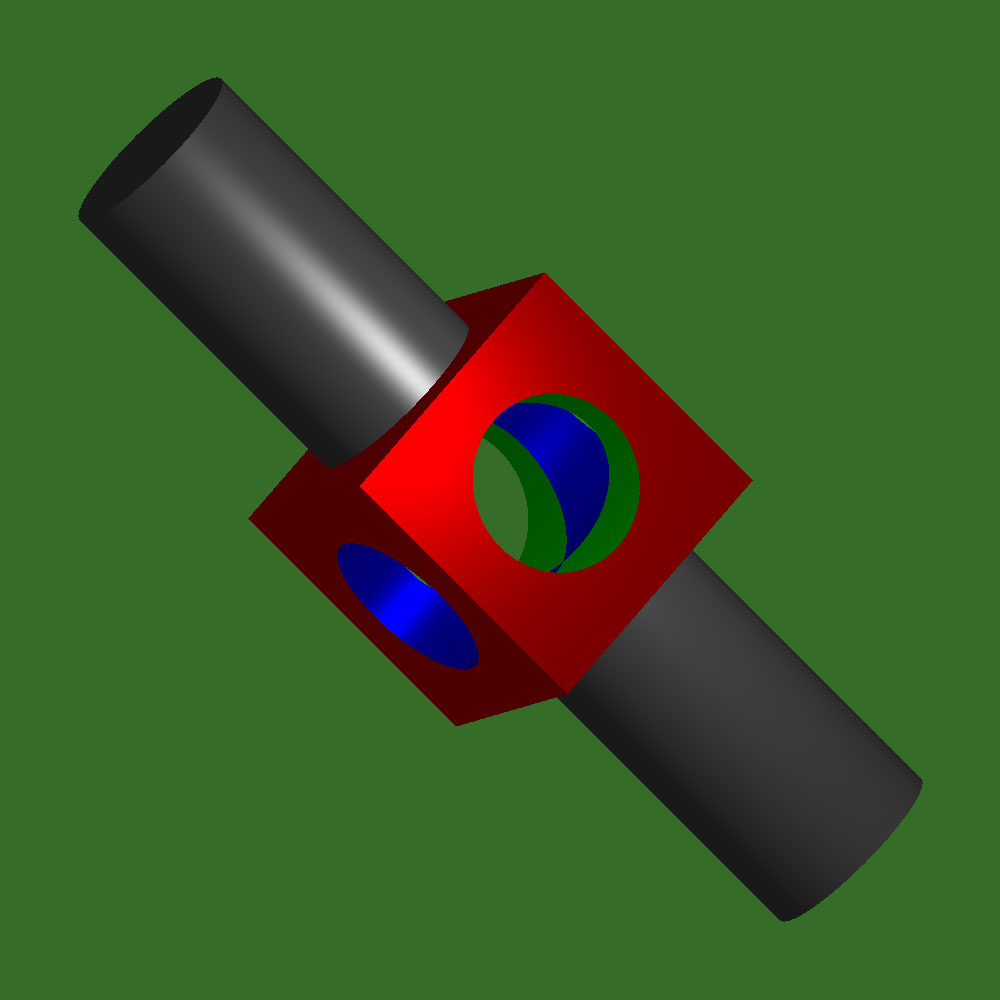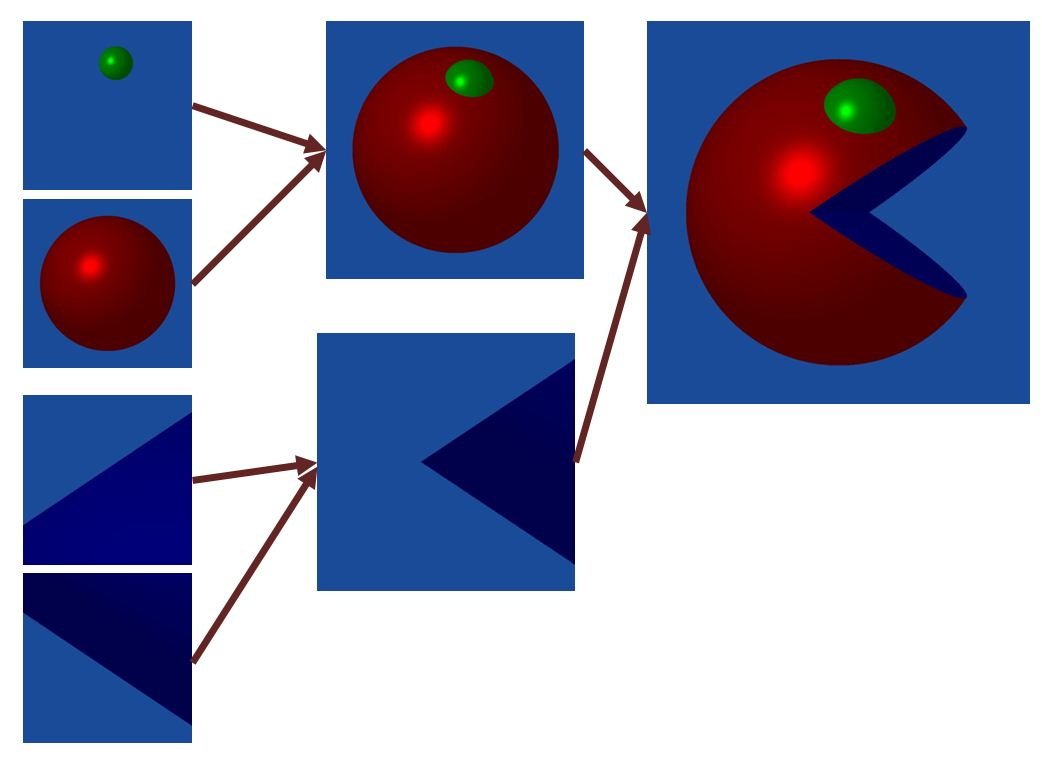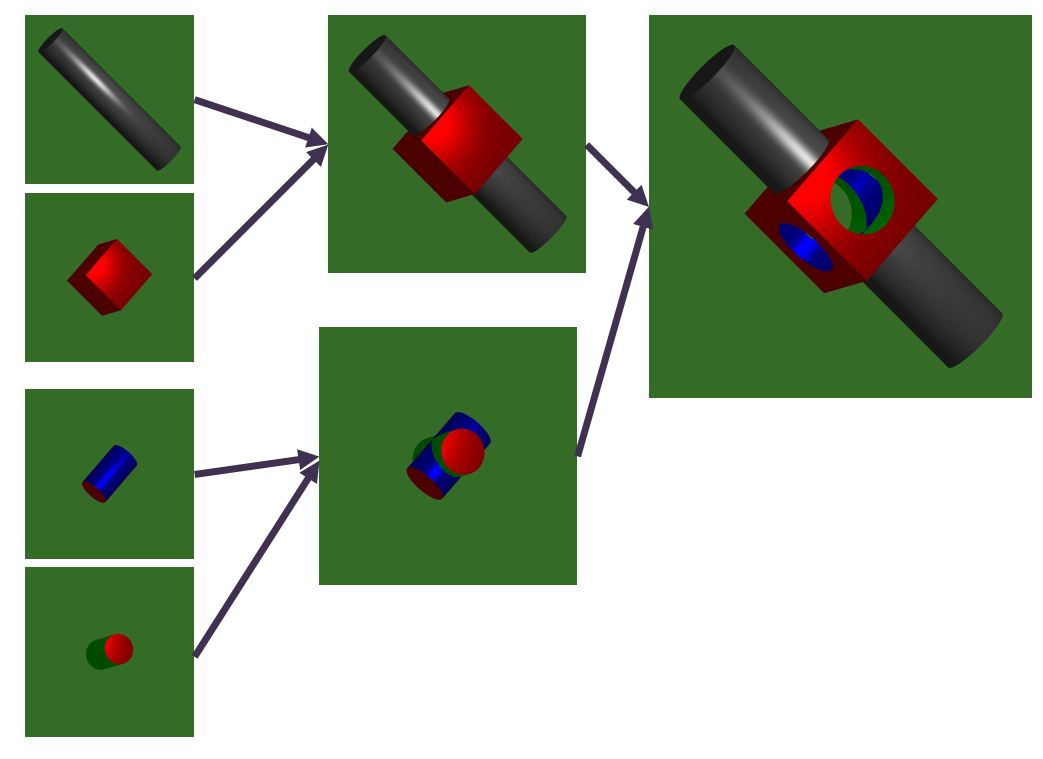# Constructive Solid Geometry

## with Ray Tracing### How it works:

Geometric primitives, such as spheres, planes, and cylinders, are combined using boolean operations like union, intersection and difference to create complex geometric shapes. A ray is cast for each pixel from the camera to the object and intersections are determined algebraically. Once the intersection is calculated, We take the closest intersection point and determine which primitive's surface falls on that point. The pixel is colored by the material properties and normal of that primitive. The object's structure is a binary tree that uses recursion to calculate intersections. Geometric primitives are leaf nodes.

### Examples: